Create a new printableGeometry
Math Worksheets

Sample - Click above to make a new math worksheet (PDF).
 Name _____________________________Date ___________________
Angle Relationships
Find the measure of the angle.

 1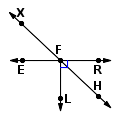m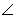HFR = _________mXFE = 432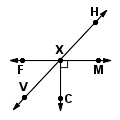mHXM = _________mVXF = 473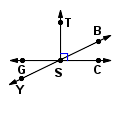mYSG = _________mYSC = 1544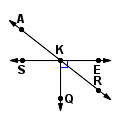mRKQ = _________mRKE = 385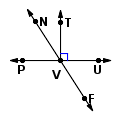mNVT = _________mNVP = 576 * This is a pre-made sheet.Use the link at the top of the page for a printable page.
 7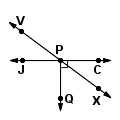mXPC = _________mVPJ = 378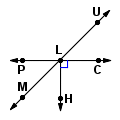mMLP = _________mPLU = 1359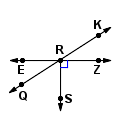mKRZ = _________mQRE = 3310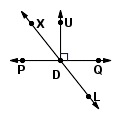mPDL = _________mLDQ = 5211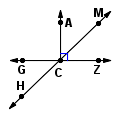mACM = _________mMCZ = 4412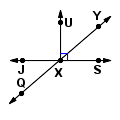mYXS = _________mQXJ = 41